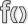# Map.PlotDirection (Civ5 API)

Jump to: navigation, search

This page is a part of the Lua and UI Reference (Civ5).This function is a member of Map. This is a static method, invoke it with a dot. Gets a plot adjacent to the map coordinates given.

# Usage

`Plot Map.PlotDirection(int x, int y, int direction)`

Returned Value

An adjacent Plot based on the coordinates and direction given.

Parameters

 x: The x coordinate of the plot of which to get the adjacent plot. y: The y coordinate of the plot of which to get the adjacent plot. direction: An integer specifying the direction of the adjacent plot relative to the coordinates given. Enumerated in DirectionType.

# Source code samples

Too many occurences. Only 50 out of 283 are listed.

Amazon_XP.lua (G&K)

`DLC/Expansion/Maps/Amazon_XP.lua`
 `0457` `local adjacentPlot = Map.PlotDirection(riverPlot:GetX(), riverPlot:GetY(), DirectionTypes.DIRECTION_EAST);` `0463` `riverPlot = Map.PlotDirection(riverPlot:GetX(), riverPlot:GetY(), DirectionTypes.DIRECTION_NORTHEAST);` `0466` `local adjacentPlot = Map.PlotDirection(riverPlot:GetX(), riverPlot:GetY(), DirectionTypes.DIRECTION_SOUTHEAST);` `0474` `riverPlot = Map.PlotDirection(startPlot:GetX(), startPlot:GetY(), DirectionTypes.DIRECTION_EAST);` `0478` `local adjacentPlot = Map.PlotDirection(riverPlot:GetX(), riverPlot:GetY(), DirectionTypes.DIRECTION_SOUTHWEST);` `0486` `riverPlot = Map.PlotDirection(startPlot:GetX(), startPlot:GetY(), DirectionTypes.DIRECTION_SOUTHWEST);` `0544` `local adjacentPlot = Map.PlotDirection(plot:GetX(), plot:GetY(), direction);` `0655` `riverPlot = Map.PlotDirection(riverPlot:GetX(), riverPlot:GetY(), DirectionTypes.DIRECTION_WEST);` `0674` `return Map.PlotDirection(riverPlotX, riverPlotY, DirectionTypes.DIRECTION_NORTHWEST);` `0678` `return Map.PlotDirection(riverPlotX, riverPlotY, DirectionTypes.DIRECTION_NORTHEAST);` `0682` `return Map.PlotDirection(riverPlotX, riverPlotY, DirectionTypes.DIRECTION_EAST);` `0686` `return Map.PlotDirection(riverPlotX, riverPlotY, DirectionTypes.DIRECTION_SOUTHWEST);` `0690` `return Map.PlotDirection(riverPlotX, riverPlotY, DirectionTypes.DIRECTION_WEST);`

AssignStartingPlots.lua

`Gameplay/Lua/AssignStartingPlots.lua`
 `5000` `local adjPlot = Map.PlotDirection(x, y, direction)` `5001` `if adjPlot:IsLake() then`

AssignStartingPlots.lua (G&K)

`DLC/Expansion/Gameplay/Lua/AssignStartingPlots.lua`
 `5194` `local adjPlot = Map.PlotDirection(x, y, direction)` `5195` `if adjPlot == nil then` `5343` `local adjPlot = Map.PlotDirection(x, y, direction)` `5344` `local plotType = adjPlot:GetPlotType();` `5421` `local adjPlot = Map.PlotDirection(x, y, direction)` `5422` `local terrainType = adjPlot:GetTerrainType();` `5516` `local adjPlot = Map.PlotDirection(x, y, direction)` `5517` `local featureType = adjPlot:GetFeatureType();` `5964` `local adjPlot = Map.PlotDirection(x, y, direction)` `5965` `if adjPlot:GetTerrainType() ~= TerrainTypes.TERRAIN_COAST then`

FeatureGenerator.lua (G&K)

`DLC/Expansion/Gameplay/Lua/FeatureGenerator.lua`
 `0367` `local adjPlot = Map.PlotDirection(x, y, direction)` `0368` `if adjPlot ~= nil then`

FractalWorld.lua

`Gameplay/Lua/FractalWorld.lua`
 `0329` `local nextPlot = Map.PlotDirection(currentX, currentY, currentDirection);`

Great_Plains.lua

`Maps/Great_Plains.lua`
 `1051` `local adjPlot = Map.PlotDirection(x, y, direction)` `1052` `plotType = adjPlot:GetPlotType();`

InGame.lua

`UI/InGame/InGame.lua`
 `0485` `local adjacentPlot = Map.PlotDirection(unitX, unitY, thisDirection);`

MapmakerUtilities.lua

`Gameplay/Lua/MapmakerUtilities.lua`
 `0319` `local testPlot = Map.PlotDirection(x, y, current_direction);` `0372` `local NEPlot = Map.PlotDirection(x, y, DirectionTypes.DIRECTION_NORTHEAST);` `0373` `local EPlot = Map.PlotDirection(x, y, DirectionTypes.DIRECTION_EAST);` `0374` `local SEPlot = Map.PlotDirection(x, y, DirectionTypes.DIRECTION_SOUTHEAST);` `0375` `local SWPlot = Map.PlotDirection(x, y, DirectionTypes.DIRECTION_SOUTHWEST);` `0376` `local WPlot = Map.PlotDirection(x, y, DirectionTypes.DIRECTION_WEST);` `0377` `local NWPlot = Map.PlotDirection(x, y, DirectionTypes.DIRECTION_NORTHWEST);`

NaturalWondersCustomMethods.lua (G&K)

`DLC/Expansion/Gameplay/Lua/NaturalWondersCustomMethods.lua`
 `0038` `local SEPlot = Map.PlotDirection(x, y, DirectionTypes.DIRECTION_SOUTHEAST)` `0039` `local southeastX = SEPlot:GetX();` `0042` `local adjPlot = Map.PlotDirection(southeastX, southeastY, direction)` `0043` `if adjPlot == nil then` `0066` `local adjPlot = Map.PlotDirection(x, y, direction)` `0067` `if adjPlot:IsWater() == false then` `0154` `local SEPlot = Map.PlotDirection(x, y, DirectionTypes.DIRECTION_SOUTHEAST)` `0155` `if not SEPlot:IsWater() then` `0167` `local adjPlot = Map.PlotDirection(southeastX, southeastY, direction)` `0168` `if adjPlot:GetTerrainType() ~= TerrainTypes.TERRAIN_COAST then` `0212` `local adjPlot = Map.PlotDirection(x, y, direction)` `0213` `if adjPlot:GetPlotType() == PlotTypes.PLOT_OCEAN then`

TurnsRemaining.lua - DLC_02 DLC

`DLC/DLC_02/Scenarios/NewWorldScenario/TurnsRemaining.lua`
 `0401` `local adjacentPlot = Map.PlotDirection(pPlot:GetX(), pPlot:GetY(), direction);`

TurnsRemaining.lua (G&K)

`DLC/Expansion/Scenarios/MedievalScenario/TurnsRemaining.lua`
 `1042` `local adjPlot = Map.PlotDirection(pPlot:GetX(), pPlot:GetY(), direction);` `1398` `local adjPlot = Map.PlotDirection(iX, iY, direction);`

WorldBuilderRandomItems.lua

`Gameplay/Lua/WorldBuilderRandomItems.lua`
 `0060` `local otherPlot = Map.PlotDirection(plotX, plotY, direction);`

The initial version of this page was created by the Civ5 API Bot, see the Civ5 API Reference FAQ. Some of the texts come from the 2kgames' wiki and most of code samples are copyrighted to Firaxis.
Functions' signatures were either copied from the 2kgames' wiki, or infered from the Lua source files and the binaries. Errors are possible.
Contributors may find help in the Contributors guide to the Civ5 API.